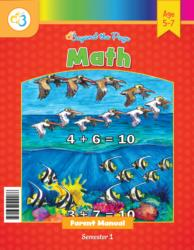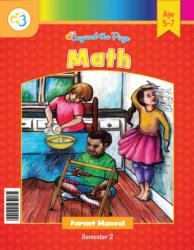# Common Core Alignment

CCSS.Math.Content.1.NBT.1 - Count to 120, starting at any number less than 120. In this range, read and write numerals and represent a number of objects with a written numeral.

## 5: MathUnit 1: Number Sense 1-20
Lesson 1: Numbers 1-5
Lesson 4: Numbers 6-10
Lesson 6: Numbers 11-15
Lesson 7: Numbers 16-20
Lesson 8: Reviewing Numbers 11-20
Final Project: My Math GameUnit 3: Addition and Subtraction to 10Unit 7: Place Value
Lesson 1: What Is the Base-10 System?
Lesson 10: More Work With 100
Lesson 11: Sequencing Numbers
Lesson 12: Comparing Numbers
Lesson 13: 10 More and 10 Less
Lesson 14: Working With Multiples of 10
Lesson 15: More Practice With 10
Lesson 2: What Is Place Value?
Lesson 3: Tens
Lesson 4: Distinguishing Tens and Ones
Lesson 5: Practice Time!
Lesson 6: More Work With Tens and Ones
Lesson 7: Building Numbers Using Tens and Ones
Lesson 8: Reaching 100!
Lesson 9: Playing With Bigger Numbers
Final Project: Place Value BINGO!